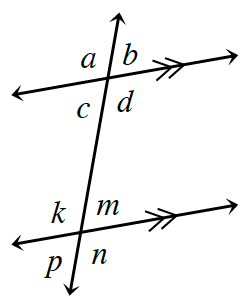### Home > INT2 > Chapter 3 > Lesson 3.1.1 > Problem3-7

3-7.

Determine the missing angle measure(s) in each problem at right using the geometric relationships shown in the diagram at right. Be sure to write down the conjecture that justifies each calculation. Each part is a separate problem.

1. If $d = 110^\circ$ and $k = 5x - 20^\circ$, write an equation and solve for $x$.

Note that $∠d$ and $∠k$ are alternate interior angles.
What is true about alternate interior angles?

2. If $b = 4x - 11^\circ$ and $n = x + 26^\circ$, write an equation and solve for $x$. Then determine the measure of $n$.

Note that $∠b$ is congruent to $∠m$ and that $∠m$ and $∠n$ are supplementary angles.
Refer to the Math Notes box in Lesson 1.3.4.